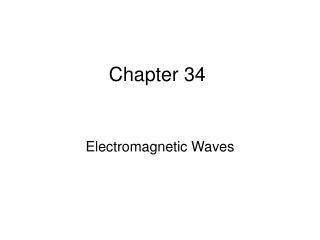DownloadDownload PresentationChapter 34

# Chapter 34

Download Presentation## Chapter 34

- - - - - - - - - - - - - - - - - - - - - - - - - - - E N D - - - - - - - - - - - - - - - - - - - - - - - - - - -
##### Presentation Transcript

1. Chapter 34 Electromagnetic Waves

2. James Clerk Maxwell • In 1865, James Clerk Maxwell provided a mathematical theory that showed a close relationship between all electric and magnetic phenomena • Maxwell’s equations also predict the existence of electromagnetic waves that propagate through space

3. Maxwell’s Equations • In his unified theory of electromagnetism, Maxwell showed that electromagnetic waves are a natural consequence of the fundamental laws expressed in these four equations:

4. Speed of Electromagnetic Waves • In empty space, q = 0 and I = 0 • The last two equations can be solved to show that the speed at which electromagnetic waves travel is the speed of light • This result led Maxwell to predict that light waves were electromagnetic waves

5. Hertz’s Confirmation of Maxwell’s Predictions • Heinrich Hertz was the first to generate and detect electromagnetic waves in a laboratory setting • The most important discoveries were in 1887 • He also showed other wave aspects of light

6. Hertz’s Experiment • An induction coil is connected to a transmitter • The transmitter consists of two spherical electrodes separated by a narrow gap

7. Hertz’s Experiment • The coil provides short voltage surges to the electrodes • As the air in the gap is ionized, it becomes a better conductor • The discharge between the electrodes exhibits an oscillatory behavior at a very high frequency • From a circuit viewpoint, this is equivalent to an LC circuit

8. Hertz’s Experiment • Sparks were induced across the gap of the receiving electrodes when the frequency of the receiver was adjusted to match that of the transmitter • In a series of other experiments, Hertz also showed that the radiation generated by this equipment exhibited wave properties • Interference, diffraction, reflection, refraction and polarization • He also measured the speed of the radiation

9. Plane EM Waves • We will assume that the vectors for the electric and magnetic fields in an EM wave have a specific space-time behavior that is consistent with Maxwell’s equations • Assume an EM wave that travels in the x direction with E and B as shown

10. Plane EM Waves • The x-direction is the direction of propagation • E is assumed to be in the y direction and B in the z direction • Waves in which the electric and magnetic fields are restricted to being parallel to a pair of perpendicular axes are said to be linearly polarized waves • We also assume that at any point in space, the magnitudes E and B of the fields depend upon x and t only

11. Rays • A ray is a line along which the wave travels • The collection of waves is called a plane wave • A surface connecting points of equal phase on all waves, called the wave front, is a geometric plane

12. Properties of EM Waves • The solutions of Maxwell’s third and fourth equations are wave-like, with both E and B satisfying a wave equation • Electromagnetic waves travel at the speed of light: • This comes from the solution of Maxwell’s equations

13. Properties of EM Waves • The components of the electric and magnetic fields of plane electromagnetic waves are perpendicular to each other and perpendicular to the direction of propagation • This can be summarized by saying that electromagnetic waves are transverse waves

14. Properties of EM Waves • The magnitudes of E and B in empty space are related by the expression • This also comes from the solution of the partial differentials obtained from Maxwell’s equations • Electromagnetic waves obey the superposition principle

15. Derivation of Speed – Some Details • From Maxwell’s equations applied to empty space, the following partial derivatives can be found: • These are in the form of a general wave equation, with • Substituting the values for μo and εo gives c = 2.99792 x 108 m/s

16. E to B Ratio – Some Details • The simplest solution to the partial differential equations is a sinusoidal wave: • E = Emax cos (kx – ωt) • B = Bmax cos (kx – ωt) • The angular wave number is k = 2π/λ • λ is the wavelength • The angular frequency is ω = 2πƒ • ƒ is the wave frequency

17. E to B Ratio – Details • The speed of the electromagnetic wave is • Taking partial derivations also gives

18. EM Wave Representation • This is a pictorial representation, at one instant, of a sinusoidal, linearly polarized plane wave moving in the x direction • E and B vary sinusoidally with x

19. Time Sequence of an EM Wave

20. Active Figure 34.3 (SLIDESHOW MODE ONLY)